# 7.11: Proficiency Exam

$$\newcommand{\vecs}{\overset { \rightharpoonup} {\mathbf{#1}} }$$ $$\newcommand{\vecd}{\overset{-\!-\!\rightharpoonup}{\vphantom{a}\smash {#1}}}$$$$\newcommand{\id}{\mathrm{id}}$$ $$\newcommand{\Span}{\mathrm{span}}$$ $$\newcommand{\kernel}{\mathrm{null}\,}$$ $$\newcommand{\range}{\mathrm{range}\,}$$ $$\newcommand{\RealPart}{\mathrm{Re}}$$ $$\newcommand{\ImaginaryPart}{\mathrm{Im}}$$ $$\newcommand{\Argument}{\mathrm{Arg}}$$ $$\newcommand{\norm}{\| #1 \|}$$ $$\newcommand{\inner}{\langle #1, #2 \rangle}$$ $$\newcommand{\Span}{\mathrm{span}}$$ $$\newcommand{\id}{\mathrm{id}}$$ $$\newcommand{\Span}{\mathrm{span}}$$ $$\newcommand{\kernel}{\mathrm{null}\,}$$ $$\newcommand{\range}{\mathrm{range}\,}$$ $$\newcommand{\RealPart}{\mathrm{Re}}$$ $$\newcommand{\ImaginaryPart}{\mathrm{Im}}$$ $$\newcommand{\Argument}{\mathrm{Arg}}$$ $$\newcommand{\norm}{\| #1 \|}$$ $$\newcommand{\inner}{\langle #1, #2 \rangle}$$ $$\newcommand{\Span}{\mathrm{span}}$$$$\newcommand{\AA}{\unicode[.8,0]{x212B}}$$

## Proficiency Exam

For the following problems, construct a coordinate system and graph the inequality.

##### Exercise $$\PageIndex{1}$$

$$−6x+4>−14$$

$$x<3$$##### Exercise $$\PageIndex{2}$$

$$−8<x+6≤−4$$

$$−14<x≤−10$$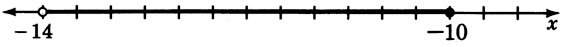##### Exercise $$\PageIndex{3}$$

Plot the ordered pairs $$(3, 1),(−2, 4),(0, 5),(−2, −2)$$.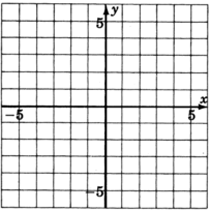##### Exercise $$\PageIndex{4}$$

As accurately as possible, label the coordinates of the points that have been plotted on the graph.$$(0,1),(3,3),(−3,0),(2,−3)$$

##### Exercise $$\PageIndex{5}$$

What is the geometric structure of the graph of all the solutions to the equation $$2y+3x=−4$$?

straight line

##### Exercise $$\PageIndex{6}$$

In what form is the linear equation in two variables $$ax+by=c$$?

general form

##### Exercise $$\PageIndex{7}$$

In what form is the linear equation in two variables $$y=mx+b$$?

slope-intercept

##### Exercise $$\PageIndex{8}$$

If an ordered pair is a solution to a linear equation in two variables, where does it lie geometrically?

It lies on the line.

##### Exercise $$\PageIndex{9}$$

Consider the graph of $$y=\dfrac{2}{7}x+16$$. If we were to place our pencil at any point on the line and then move it horizontally $$7$$ units to the right, how many units and in what direction would we have to move our pencil to get back on the line?

$$2$$ units up

For the following problems, find the slope, if it exists, of the line containing the following points.

##### Exercise $$\PageIndex{10}$$

(−6, −1) and (0, 8)

$$\dfrac{3}{2}$$

##### Exercise $$\PageIndex{11}$$

(−2, −8) and (−2, 10)

no slope; vertical line at $$x=−2$$

##### Exercise $$\PageIndex{12}$$

Determine the slope and $$y$$−intercept of the line $$3y+2x+1=0$$.

slope = $$-\dfrac{2}{3}$$, $$y$$-intercept is $$(0, -\dfrac{1}{3})$$

##### Exercise $$\PageIndex{13}$$

As we look at a graph left to right, do lines with a positive slope rise or decline?

rise

For the following problems, find the equation of the line using the information provided. Write the equation in slope-intercept form

##### Exercise $$\PageIndex{14}$$

Slope = $$4$$, $$y$$-intercept = $$−3$$.

$$y=4x−3$$

##### Exercise $$\PageIndex{15}$$

Slope = $$-\dfrac{3}{2}$$, $$y$$-intercept = $$\dfrac{4}{3}$$.

$$y = -\dfrac{3}{2}x + \dfrac{4}{3}$$

##### Exercise $$\PageIndex{16}$$

Slope = $$\dfrac{2}{3}$$, passes through $$(-1, 2)$$

$$y = \dfrac{2}{3}x + \dfrac{8}{3}$$

##### Exercise $$\PageIndex{17}$$

Slope = $$7$$, passes through $$(0, 0)$$

$$y=7x$$

##### Exercise $$\PageIndex{18}$$

Passes through the points $$(5, 2)$$ and $$(2, 1)$$.

$$y = \dfrac{1}{3}x + \dfrac{1}{3}$$

For the following problems, graph the equation of inequality.

##### Exercise $$\PageIndex{19}$$

$$y = \dfrac{1}{3}x - 2$$$$y = \dfrac{1}{3}x - 2$$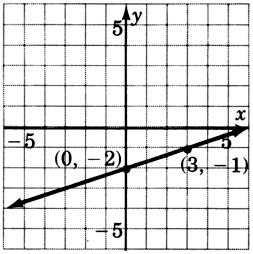##### Exercise $$\PageIndex{20}$$

$$5y−2x+15=0$$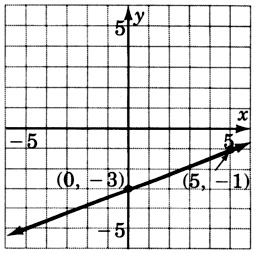##### Exercise $$\PageIndex{21}$$

$$4(x+y)=8$$$$4(x+y)=8$$##### Exercise $$\PageIndex{22}$$

$$\dfrac{3}{2}y + 2 = 0$$##### Exercise $$\PageIndex{23}$$

$$x=−2$$$$x=−2$$##### Exercise $$\PageIndex{24}$$

$$2x+3y>6$$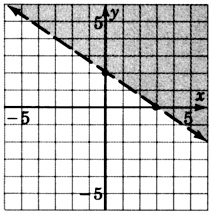##### Exercise $$\PageIndex{25}$$

Reading only from the graph, determine the equation of the line.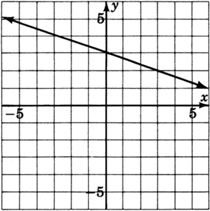$$y = -\dfrac{1}{3}x + 3$$